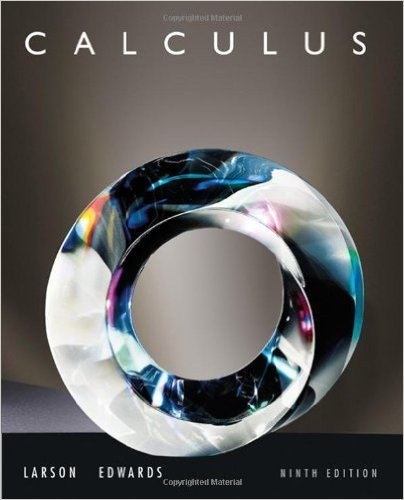×
×

# Solutions for Chapter 2.5: Implicit Differentiation## Full solutions for Calculus | 9th Edition

ISBN: 9780547167022Solutions for Chapter 2.5: Implicit Differentiation

Solutions for Chapter 2.5
4 5 0 321 Reviews
20
3
##### ISBN: 9780547167022

Calculus was written by and is associated to the ISBN: 9780547167022. Chapter 2.5: Implicit Differentiation includes 81 full step-by-step solutions. This expansive textbook survival guide covers the following chapters and their solutions. This textbook survival guide was created for the textbook: Calculus , edition: 9. Since 81 problems in chapter 2.5: Implicit Differentiation have been answered, more than 64068 students have viewed full step-by-step solutions from this chapter.

Key Calculus Terms and definitions covered in this textbook

P(A or B) = P(A) + P(B) - P(A and B). If A and B are mutually exclusive events, then P(A or B) = P(A) + P(B)

• Additive inverse of a real number

The opposite of b , or -b

• Central angle

An angle whose vertex is the center of a circle

• Convergence of a series

A series aqk=1 ak converges to a sum S if imn: q ank=1ak = S

• Difference identity

An identity involving a trigonometric function of u - v

• Double-blind experiment

A blind experiment in which the researcher gathering data from the subjects is not told which subjects have received which treatment

• First quartile

See Quartile.

• Geometric series

A series whose terms form a geometric sequence.

• Imaginary unit

The complex number.

• Invertible linear system

A system of n linear equations in n variables whose coefficient matrix has a nonzero determinant.

• Range (in statistics)

The difference between the greatest and least values in a data set.

• Real axis

See Complex plane.

• Recursively defined sequence

A sequence defined by giving the first term (or the first few terms) along with a procedure for finding the subsequent terms.

• Replication

The principle of experimental design that minimizes the effects of chance variation by repeating the experiment multiple times.

• Sum of complex numbers

(a + bi) + (c + di) = (a + c) + (b + d)i

• Variable

A letter that represents an unspecified number.

• Vertex of a cone

See Right circular cone.

• Vertical line

x = a.

• Zero of a function

A value in the domain of a function that makes the function value zero.

• Zero vector

The vector <0,0> or <0,0,0>.

×